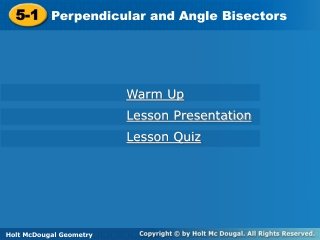DownloadDownload Presentation5-1

# 5-1

Télécharger la présentation## 5-1

- - - - - - - - - - - - - - - - - - - - - - - - - - - E N D - - - - - - - - - - - - - - - - - - - - - - - - - - -
##### Presentation Transcript

1. 5-1 Perpendicular and Angle Bisectors Warm Up Lesson Presentation Lesson Quiz Holt McDougal Geometry Holt Geometry

2. Warm Up 1. Find the midpoint and slope of the segment (2, 8) and (–4, 6).

3. Objectives Prove and apply theorems about perpendicular bisectors. Prove and apply theorems about angle bisectors.

4. When a point is the same distance from two or more objects, the point is said to be equidistantfrom the objects. Triangle congruence theorems can be used to prove theorems about equidistant points.

5. Example 1A: Applying the Perpendicular Bisector Theorem and Its Converse Find each measure. MN MN = LN  Bisector Thm. MN = 2.6 Substitute 2.6 for LN.

6. Since AB = AC and , is the perpendicular bisector of by the Converse of the Perpendicular Bisector Theorem. Example 1B: Applying the Perpendicular Bisector Theorem and Its Converse Find each measure. BC BC = 2CD Def. of seg. bisector. BC = 2(12) = 24 Substitute 12 for CD.

7. Example 1C: Applying the Perpendicular Bisector Theorem and Its Converse Find each measure. TU TU = UV  Bisector Thm. 3x + 9 = 7x – 17 Substitute the given values. 9 = 4x – 17 Subtract 3x from both sides. 26 = 4x Add 17 to both sides. 6.5 = x Divide both sides by 4. So TU = 3(6.5) + 9 = 28.5.

8. Since DG = EG and , is the perpendicular bisector of by the Converse of the Perpendicular Bisector Theorem. Check It Out! Example 1b Find the measure. Given that DE = 20.8, DG = 36.4, and EG =36.4, find EF. DE = 2EF Def. of seg. bisector. 20.8 = 2EF Substitute 20.8 for DE. 10.4 = EF Divide both sides by 2.

9. Example 2A: Applying the Angle Bisector Theorem Find the measure. BC BC = DC  Bisector Thm. BC = 7.2 Substitute 7.2 for DC.

10. Since EH = GH, and , bisects EFGby the Converse of the Angle Bisector Theorem. Example 2B: Applying the Angle Bisector Theorem Find the measure. mEFH, given that mEFG = 50°. Def. of  bisector Substitute 50° for mEFG.

11. Since, JM = LM, and , bisects JKL by the Converse of the Angle Bisector Theorem. Example 2C: Applying the Angle Bisector Theorem Find mMKL. mMKL = mJKM Def. of  bisector 3a + 20 = 2a + 26 Substitute the given values. a + 20 = 26 Subtract 2a from both sides. a= 6 Subtract 20 from both sides. So mMKL = [2(6) + 26]° = 38°

12. Check It Out! Example 2a Given that YW bisects XYZ and WZ = 3.05, find WX. WX = WZ  Bisector Thm. WX = 3.05 Substitute 3.05 for WZ. So WX = 3.05

13. Check It Out! Example 2b Given that mWYZ = 63°, XW = 5.7, and ZW = 5.7, find mXYZ. mWYZ + mWYX = mXYZ  Bisector Thm. mWYZ = mWYX Substitute m WYZ for mWYX . mWYZ +mWYZ= mXYZ Simplify. 2mWYZ = mXYZ 2(63°) = mXYZ Substitute 63° for mWYZ . 126° = mXYZ Simplfiy.

14. Use the diagram for Items 3–4. 3. Given that FH is the perpendicular bisector of EG, EF = 4y – 3, and FG = 6y – 37, find FG. 4. Given that EF = 10.6, EH = 4.3, and FG = 10.6, find EG. Lesson Quiz: Part I Use the diagram for Items 1–2. 1. Given that mABD = 16°, find mABC. 2. Given that mABD = (2x + 12)° and mCBD = (6x – 18)°, find mABC. 32° 54° 65 8.6